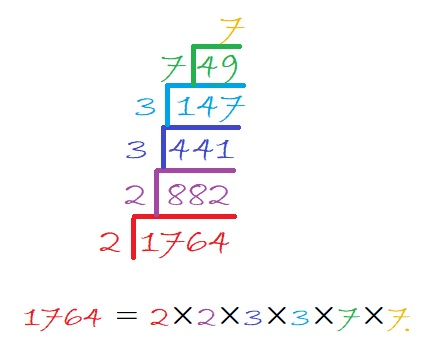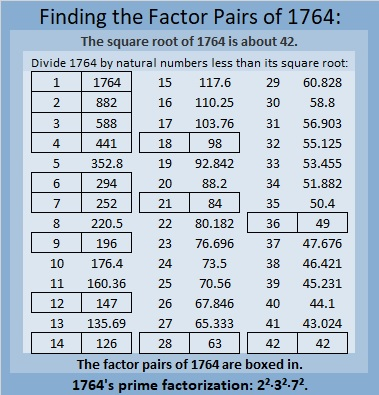# 1764 Perfect Squares Are Amazing!

Contents

### Today’s Puzzle:

1764 is a perfect square, and its last two digits are a perfect square, too. The same thing was true of the last perfect square, 1681. And it will be true of the next perfect square, 1849. These perfect squares got me curious about perfect squares in general, and I noticed something. Can you notice it, too?

Look at this graphic of perfect squares. What do you notice? What do you wonder?I have known for years that the last digits of perfect squares follow a palindromic
0-1-4-9-6-5-6-9-4-1-0 pattern. The first time the backward repeating happens is at 5¹.

I had no idea that the last two digits followed a palindromic pattern, too! Notice that the first time the backward repeating of the last two digits happens is at (5²)² or 25². It also happens at 75², 125², and so forth. The forward repeating happens at 0², 50², 100², and so forth. Note that (-n)² = n², so this pattern goes on in both directions FOREVER!

Also if the last two digits of a number aren’t either 00, 25, or (even number)1, (even number)4, (even number)9,  or (odd number)6, then no way is it a perfect square!

I wondered if the last three digits would backward repeat at (5³)² or 125². It doesn’t. 🙁
But at 250², it DOES! Rather than make a graphic with all 250 rows, I just made one with the last 25 rows:Will the last FOUR numbers start backward repeating at 2500²? I know the answer, but perhaps you would like to find out for yourself!

### Factors of 1764:• 1764 is a composite number and a perfect square.
• Prime factorization: 1764 = 2 × 2 × 3 × 3 × 7 × 7, which can be written 1764 = 2² × 3² × 7².
• 1764 has at least one exponent greater than 1 in its prime factorization so √1764 can be simplified. √1764 = √(42 × 42) = 42.
• The exponents in the prime factorization are 2, 2, and 2. Adding one to each exponent and multiplying we get (2 + 1)(2 + 1)(2 + 1) = 3 × 3 × 3 = 27. Therefore 1764 has exactly 27 factors. (Only perfect squares can have an odd number of factors.)
• The factors of 1764 are outlined with their factor pair partners in the graphic below.### More About the Number 1764:

Square number, 1764,  looks square in some other bases, too:
It’s 900 in base 14 because 9(14²) + 0(14) + 0(1) = 1764,
484 in base 20 because 4(20²) + 8(20) + 4(1) = 1764,
400 in base 21 because 4(21²) + 0(21) + 0(1) = 1764,
169 in base 39 because 1(39²) + 6(39) + 9(1) = 1764,
144 in base 40 because 1(40²) + 4(40) + 4(1) = 1764,
121 in base 41 because 1(41²) + 2(41) + 1(1) = 1764, and
100 in base 42 because 1(42²) + 0(42) + 0(1) = 1764.

## 2 thoughts on “1764 Perfect Squares Are Amazing!”

1.Charles Sanders

(4n+1)^2=4n^2+8n+1, (4n+3)^2=4n^2+24n+9, (2n)^2=4n^2, 2^2=4. Is this what you mean?

•ivasallay

Thank you very much for your feedback! All those are true mathematical facts, but they aren’t what I was thinking about. I am simply amazed that infinitely in both directions for winding columns of 25, the last TWO digits in each row of square numbers are the same. Also, infinitely in both directions for winding columns of 250, the last THREE digits in each row of square numbers are the same, and infinitely in both directions for winding columns of 2500, the last FOUR digits in each row of square numbers are the same. I could go on and on.

This site uses Akismet to reduce spam. Learn how your comment data is processed.